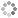# PROWAREtecharticles » current » javascript » check-number-for-power-of-2

## JavaScript: Is a Number a Power of Two (2)

How to check or determine if a number has a base of 2 without using modulo operations for maximum performance.

See how to find a number with a base of 2 in C#.

### Solution One: Use log2

The simplest way to determine if a number is a power of two is to use log2 and it will return an integer if so.

### Solution Two: Use Brute Force

Simply keep dividing the number by 2 and then if the first bit is not turned on, and the number is not itself 1, then then the number is a power of 2 (base 2).

In other words, divide the number by two (2) iteratively until it becomes one (1). If the number becomes 1 then it is a power of 2 (base 2).

``````
function isBase2 (num) {
if (num == 0) {
return false;
}
while (num != 1) {
num /= 2;
if ((num & 1) != 0 && num != 1) {
return false;
}
}
return true;
}
alert("0=" + isBase2(0) + " 1=" + isBase2(1) + " 2=" + isBase2(2) + " 3=" + isBase2(3) + " 4=" + isBase2(4) + " 10=" + isBase2(10) + " 12=" + isBase2(12) + " 16=" + isBase2(16));
``````

### Solution Three: Bit-wise Operations

Bit-wise operations are very fast and this should be the preferred method.

If the number is a power of two then the number AND (&) the same number minus one (1) will not be zero (0) unless the number itself is zero.

``````
function isBase2 (num) {
return (num & (num - 1)) == 0 && num != 0;
}
alert("0=" + isBase2(0) + " 1=" + isBase2(1) + " 2=" + isBase2(2) + " 3=" + isBase2(3) + " 4=" + isBase2(4) + " 10=" + isBase2(10) + " 12=" + isBase2(12) + " 16=" + isBase2(16));
``````This site uses cookies. Cookies are simple text files stored on the user's computer. They are used for adding features and security to this site. Read the privacy policy.